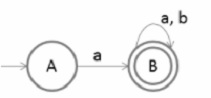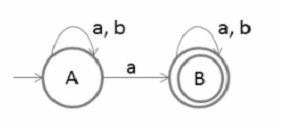# How to convert FA to Right Linear Regular Grammar?

A grammar with at most one variable at the right side of production is called linear grammar.

S→aSb/ε

S→Ab

A→aAb

A→ε

## Right Linear Grammar

A grammar is right linear grammar where all the non terminals in the right hand sides are at the right end.

For example,

S->aS/ε

## Algorithm for conversion

The algorithm to convert the finite automata (FA) to the right linear grammar is as follows −

Step 1 − Begin the process from the start state.

Step 2 − Repeat the process for each state.

Step 3 − Write the production as the output followed by the state on which the transition is going.

Step 4 − And at last, add € (epsilon) to end the derivation.

## Example 1

Let’s consider a Finite automaton (FA) as given below −Pick the start state A and output is on symbol ‘a’ going to state B

A→aB

Now we will pick state B and then we will go on each output

i.e B→aB

B→bB

B→ε

Therefore,

Final right linear grammar is as follows −

A→aB

B→aB/bB/ε

## Example 2Start with state A which is an initial state the output on symbol ‘a’ goes to A and B and the output on symbol ‘b’ goes to A. Now the production rule of right linear grammar is −

A→aA/bA/aB

Now pick state B and then go on each output the right linear grammar is −

B→aB/bB/ε

The final right linear grammar for the given finite automata is −

A→aA/bA/aB

B→aB/bB/ε

Updated on: 12-Jun-2021

3K+ Views